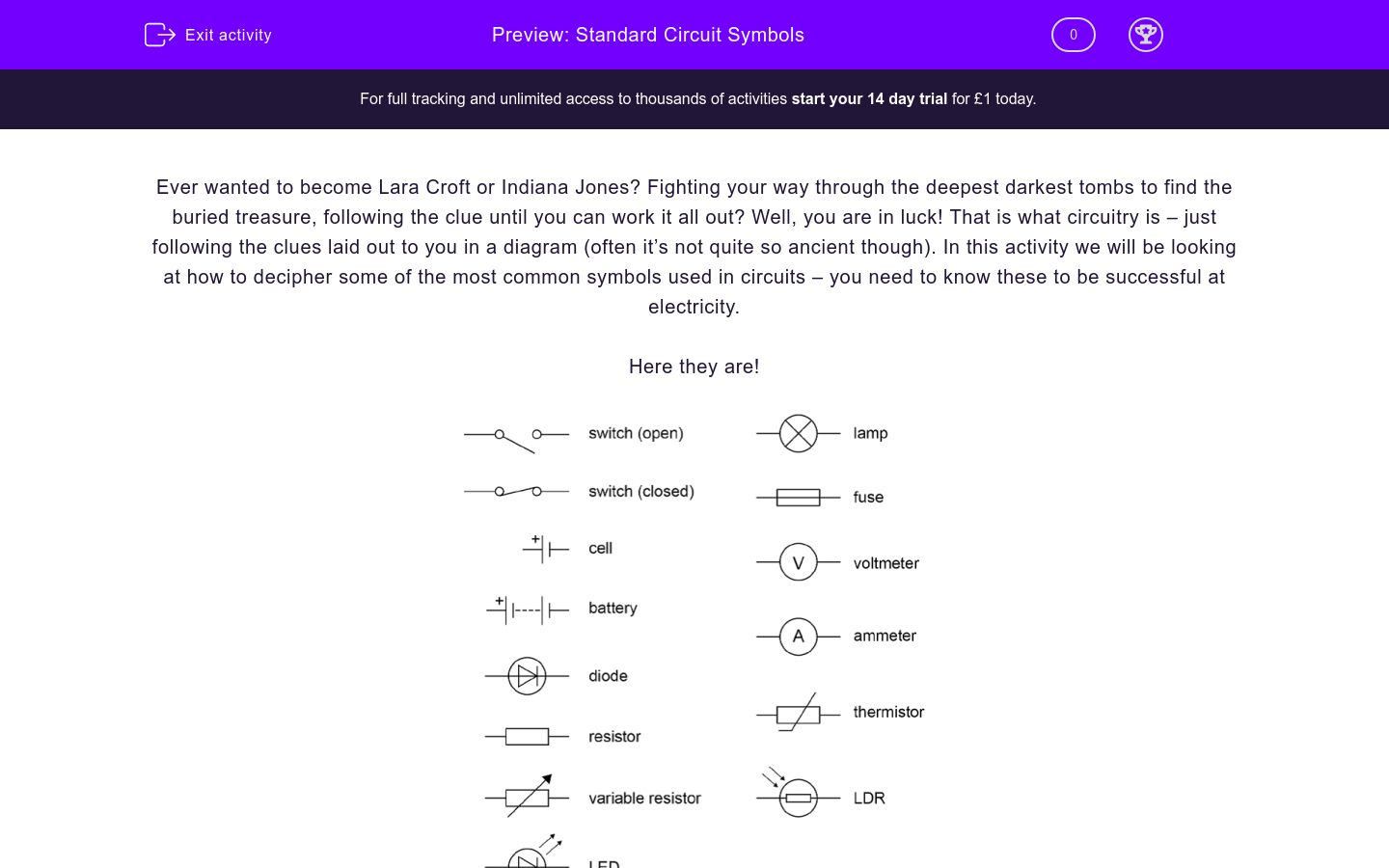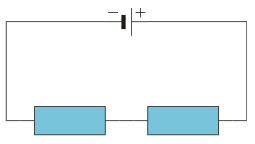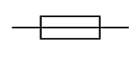# Standard circuit symbols

In this worksheet, students will look at the standard circuit symbols and how they fit into a circuit.Key stage:  KS 4

Curriculum topic:  Physics: Electricity

Curriculum subtopic:  Circuit Diagrams

Difficulty level:### QUESTION 1 of 10

Ever wanted to become Lara Croft or Indiana Jones? Fighting your way through the deepest darkest tombs to find the buried treasure, following the clue until you can work it all out? Well, you are in luck! That is what circuitry is – just following the clues laid out to you in a diagram (often it’s not quite so ancient though). In this activity we will be looking at how to decipher some of the most common symbols used in circuits – you need to know these to be successful at electricity.

Here they are!There are some important things to remember about each of these symbols though:

Ammeters measure current in amperes or amps (A). Ammeters are always connected in series (in line with the other equipment). Voltmeters measure voltage, which is the potential difference (difference in electrical energy between two points) and drives the current flow. Voltage is measured in volts (V). A voltmeter is always connected to a circuit in parallel to the component with the voltage to be measured. Electricity doesn’t flow through a voltmeter and so it will break the circuit if you put in in series.

Identify whether this circuit is series or parallel:Series

Parallel

Identify whether this circuit is series or parallel:Series

Parallel

What is this symbol representing?Diode

Voltmeter

Bulb

Ammeter

What is this symbol?Fuse

Resistor

Voltmeter

What is this symbol?Voltmeter

Lamp

Resistor

What is this symbol?Open switch

Closed switch

Switch

How is a voltmeter connected to a circuit?

In series

In parallel

What circuit component is shown in this diagram?Battery

Cell

Motor

What is this symbol?Diode

Closed switch

Resistor

What is this symbol?Cell

Switch

Battery

• Question 1

Identify whether this circuit is series or parallel:CORRECT ANSWER
Series
EDDIE SAYS
This is a series circuit, because the components are connected in one loop.
• Question 2

Identify whether this circuit is series or parallel:CORRECT ANSWER
Parallel
EDDIE SAYS
This circuit is parallel, because there are two loops.
• Question 3

What is this symbol representing?CORRECT ANSWER
Ammeter
EDDIE SAYS
The hit here is the A - it tells you what letter the name starts with.
• Question 4

What is this symbol?CORRECT ANSWER
Fuse
EDDIE SAYS
Fuse looks like a resistor but has a line going through it.
• Question 5

What is this symbol?CORRECT ANSWER
Lamp
EDDIE SAYS
Lamps have the x in them - I don't know why. X marks the spot of the light?
• Question 6

What is this symbol?CORRECT ANSWER
Open switch
EDDIE SAYS
When there is a break (bit's don't touch) then it means that the circuit doesn't work. Circuits don't work when there is an open switch in them.
• Question 7

How is a voltmeter connected to a circuit?

CORRECT ANSWER
In parallel
EDDIE SAYS
A voltmeter is always connected in parallel.
• Question 8

What circuit component is shown in this diagram?CORRECT ANSWER
Battery
EDDIE SAYS
It is a battery made of many cells put together.
• Question 9

What is this symbol?CORRECT ANSWER
Resistor
EDDIE SAYS
This is a resistor. It is just a square that current travels though - like the image!
• Question 10

What is this symbol?CORRECT ANSWER
Cell
EDDIE SAYS
A cell is one of these - a battery is multiple of these all put together.
---- OR ----

Sign up for a £1 trial so you can track and measure your child's progress on this activity.

### What is EdPlace?

We're your National Curriculum aligned online education content provider helping each child succeed in English, maths and science from year 1 to GCSE. With an EdPlace account you’ll be able to track and measure progress, helping each child achieve their best. We build confidence and attainment by personalising each child’s learning at a level that suits them.

Start your £1 trial